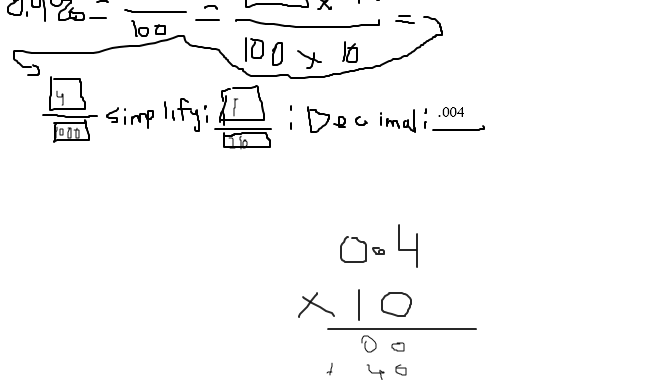Saltear al contenido principal# Comunicación asertiva para incentivar al estudiante: Tutoría de Matemáticas

Esta sesión ilustra muy bien cómo es que una comunicación correcta ayuda a que el estudiante tenga confianza en él mismo y, de ese modo, pueda trabajar en un ambiente más adecuado y contento.

Generalmente, entre más cómodo se sienta el alumno, será mucho más fácil que entienda los conceptos y si no logra entender alguno, facilita mucho el intentar otro enfoque sin que exista frustración.Student Write each percent as a fraction and as a decimal. Write fractions in simplest form.

Tutor Hi! How are you today?

Student hello!

Tutor Welcome! Let’s get started.

Student alrighty

Tutor So, your problem is 0.4 percent right?

Student correcto

Tutor Awesome! So, let’s try this together!

Student k !

Tutor Do you remember what the word «percent» means?

Student yes

Tutor Ok What would be your definition?

Student i think its stands for over 100

Tutor Exactly! So, «0.4 percent» would be the same as «0.4 over 100»

Student alrighty

Tutor So, we can actually put that on the board

Student ok oops messed up a bit on the 4

Tutor It’s ok

Student lol

Tutor So, based on the drawing, what do you think we should do next?

Student simplify?

Tutor Oh almost Let’s take a look at this part!

Student okey dokey

Tutor In that part, we’re asked to multiply by 10, right?

Student yep

Tutor Why do you think we multiply times 10?

Student i am not sure

Tutor Oh, the 0.4 was a decimal, so to make it become a whole number we would multiply by 10 That way it’s easier!

Student oh i see!

Tutor So, we’re multiplying 0.4 by 10 Let’s write 0.4 here

Student ok

Tutor Ok! Awesome! So, now, let’s multiply What do you get when you do 0.4 times 10?

Student alrighty want me to do it on the board?

Tutor Sure !

Student 4

Tutor Yup! So, let’s write 4 here

Student ok!

Tutor Ok good! So, now, let’s multiply 100 x 10

Student ok! 1000

Tutor Yup! so, 1000 goes in this box

Student ok!

Tutor Ok! Now, we have our fraction 4/1000 and the next step says «simplify» . How would you simplify?

Student by 4?

Tutor Ok, that works !

Student 1/250?

Tutor Perfect! So, let’s write that here!

Student OK! sorry i made that a small box

Tutor It’s ok So, one more step to go.

Student lol ok!

Student as ready as i could ever be

Tutor Ok! So, from the fractions we got here, we have to find the equivalent decimal Do you have any ideas on how to do that?

Student um…. no

Tutor Oh ok It’s really really easy. Let’s take at this 4/1000

Student ya

Tutor Isn’t that fraction also called «four thousandths» ?

Student yes

Tutor And how do you write «four thousandths» as a decimal?

Student ohhhhh!!!!!! i see .004

Tutor You got it! You got it! You got it! You got it! Awesome!

Student Tutor Perfect job today! Do you have any questions about anything we went over today?

Student no but thanks for the help!

Tutor No problem! 🙂 I hope you enjoyed it! Bye and have a great rest of the day

Student bye!

Colaborador: Juan Pablo Rodríguez, Tutor Mexicano de Matemáticas

Volver arriba